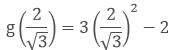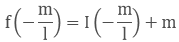×#### Thank you for registering.

One of our academic counsellors will contact you within 1 working day.

Click to Chat

1800-1023-196

+91-120-4616500

CART 0

• 0

MY CART (5)

Use Coupon: CART20 and get 20% off on all online Study Material

ITEM
DETAILS
MRP
DISCOUNT
FINAL PRICE
Total Price: Rs.

There are no items in this cart.
Continue Shopping• Complete JEE Main/Advanced Course and Test Series
• OFFERED PRICE: Rs. 15,900
• View Details

```Chapter 6: Factorization of Polynomials Exercise – 6.2

Question: 1

If f(x) = 2x3 − 13x2 + 17x + 12, Find

1. f(2)

2. f(-3)

3. f(0)

Solution:

The given polynomial is f(x) = 2x3 − 13x2 + 17x + 12

1. f(2)

we need to substitute the '2' in f(x)

f(2) = 2(2)3 − 13(2)2 + 17(2) + 12

= (2 * 8) - (13 * 4) + (17 * 2) + 12

= 16 - 52 + 34 + 12

= 10

therefore f(2) = 10

2. f(-3)

we need to substitute the '(-3)' in f(x)

f(-3) = 2(−3)3 − 13(−3)2 + 17(−3) + 12

= (2 * -27) - (13 * 9) - ( 17 * 3) + 12

= – 54 - 117 - 51 + 12

= -210

therefore f(-3) = -210

3. f(0)

we need to substitute the '(0)' in f(x)

f(0) = 2(0)3 − 13(0)2 + 17(0) + 12

= (2 * 0) - ( 13 * 0) + (17 * 0) + 12

= 0 - 0 + 0 + 12

= 12

therefore f(0) = 12

Question: 2

1. f(x) = 3x + 1, x = −1/3

2. f(x) = x2 − 1, x = (1,−1)4. p(x) = x3 − 6x2 + 11x − 6, x = 1, 2, 3

5. f(x) = 5x − π, x = 4/5

6. f(x) = x2, x = 0

7. f(x) = lx + m, x = - m/l

8. f(x) = 2x + 1, x = 1/2

Solution:

1. f(x) = 3x + 1, x = −1/3

we know that ,

f(x) = 3x + 1

substitute x = −1/3 in f(x)

f( −1/3) = 3(−1/3) + 1

= -1 + 1

= 0

Since, the result is 0 x = −1/3 is the root of 3x + 1

2. f(x) = x2 − 1, x = (1,−1)

we know that,

f(x) = x2 - 1

Given that x = (1, -1)

substitute x = 1 in f(x)

f(1) = 12 - 1

= 1 - 1

= 0

Now, substitute x = (-1) in f(x)

f(-1) = (−1)2 - 1

= 1 - 1

= 0

Since, the results when x = (1, -1) are 0 they are the roots of the polynomial f(x) = x2 - 1We know that

g(x) = 3x2 − 2

Given that,Substitute x = 2/√3 in g(x)= 3(4/3) - 2

= 4 - 2

= 2 ≠ 0

Now, Substitute x = - 2/√3 in g(x)= 3(4/3) - 2

= 4 - 2

= 2 ≠ 0

Since, the results whenare not 0, they are roots of 3x2 − 2

4. p(x) = x3 − 6x2 + 11x − 6, x = 1, 2, 3

We know that,

p(x) = x3 − 6x2 + 11x − 6

given that the values of x are 1, 2 , 3

substitute x = 1 in p(x)

p(1) = 13 - 6(1)2 + 11(1) − 6

= 1 - (6 * 1) + 11 - 6

= 1 - 6 + 11 - 6

= 0

Now, substitute x = 2 in p(x)

P(2) = 23 - 6(2)2 + 11(2) − 6

= (2 * 3) - (6 * 4) + (11 * 2) - 6

= 8 - 24 - 22 - 6

= 0

Now, substitute x = 3 in p(x)

P(3) = 33 - 6(3)2 + 11(3) − 6

= (3 * 3) - (6 * 9) + (11 * 3) - 6

= 27 - 54 + 33 - 6

= 0

Since, the result is 0 for x = 1, 2, 3 these are the roots of x3 − 6x2 + 11x − 6

(5) f(x) = 5x − π, x = 4/5

we know that,

f(x) = 5x − π

Given that, x = 4/5

Substitute the value of x in f(x)

f(4/5) = 5(4/5) - π

= 4 - π

≠ 0

Since, the result is not equal to zero, x = 4/5 is not the root of the polynomial 5x - π

(6) f(x) = x2, x = 0

we know that , f(x) = x2

Given that value of x is '0'

Substitute the value of x in f(x)

f(0) = 02

= 0

Since, the result is zero, x = 0 is the root of x2

7. f(x) = lx + m, x = - m/l

We know that,

f(x) = lx + m

Given, that x = - m/l

Substitute the value of x in f(x)= - m + m

= 0

Since, the result is 0, x = - m/l is the root of lx + m

(8) f(x) = 2x + 1, x = ½

We know that,

f(x) = 2x + 1

Given that x = 1/2

Substitute the value of x and f(x)

f(1/2) = 2(1/2) + 1

= 1 + 1

= 2 ≠ 0

Since, the result is not equal to zero

x = 1/2 is the root of 2x + 1

Question: 3

If x = 2 is a root of the polynomial f(x) = 2x2 − 3x + 7a, Find the value of a

Solution:

We know that, f(x) = 2x2 − 3x + 7a

Given that x = 2 is the root of f(x)

Substitute the value of x in f(x)

f(2) = 2(2)2 - 3(2) + 7a

= (2 * 4) - 6 + 7a

= 8 - 6 + 7a

= 7a + 2

Now, equate 7a + 2 to zero

⟹ 7a + 2 = 0

⟹ 7a = - 2

⟹ a = - 27

The value of a = - 2/7

Question: 4

If x = −1/2 is zero of the polynomial p(x) = 8x3 − ax2 − x + 2, Find the value of a

Solution:

We know that, p(x) = 8x3 − ax2 − x + 2

Given that the value of x = -1/2

Substitute the value of x in f(x)

p(-1/2) = 8(-1/2)3 - a(-1/2)2 - (-1/2) + 2

= - 8(1/8) - a(1/4) + 1/2 + 2

= -1 - (a/4 + 1/2 + 2)

= 1 - (a/4 + 1/2)

= 3/2 − a/4

To, find the value of a, equate p(-1/2) to zero

p(-1/2) = 0

3/2 - a/4 = 0

On taking L.C.M⟹ 6 - a = 0

⟹ a = 6

Question: 5

If x = 0 and x = -1 are the roots of the polynomial f(x) = 2x3 − 3x2 + ax + b, Find the of a and b.

Solution:

We know that, f(x) = 2x3−3x2 + ax + b

Given, the values of x are 0 and -1

Substitute x = 0 in f(x)

f(0) = 2(0)3 - 3(0)2 + a(0) + b

= 0 - 0 + 0 + b

= b .... 1

Substitute x = (-1) in f(x)

f(-1) = 2(−1)3 - 3(−1)2 + a(−1) + b

= -2 - 3 - a + b

= – 5 - a + b ..... 2

We need to equate equations 1 and 2 to zero

b = 0 and – 5 – a + b = 0

since, the value of b is zero

substitute b = 0 in equation 2

⟹ – 5 – a = – b

⟹ – 5 – a = 0

a = – 5

the values of a and b are - 5 and 0 respectively

Question: 6

Find the integral roots of the polynomial f(x) = x3 + 6x2 + 11x + 6

Solution:

Given, that f(x) = x3 + 6x2 + 11x + 6

Clearly we can say that, the polynomial f(x) with an integer coefficient and the highest degree term coefficient which is known as leading factor is 1.

So, the roots of f(x) are limited to integer factor of 6, they are ±1, ± 2, ± 3, ± 6

Let x = -1

f(-1) = (−1)3 + 6(−1)2 + 11(−1) + 6

= -1 + 6 -11 + 6

= 0

Let x = – 2

f(-2) = (−2)3 + 6(−2)2 + 11(−2) + 6

= – 8 – (6 * 4) - 22 + 6

= – 8 + 24 - 22 + 6

= 0

Let x = – 3

f(-3) = (-3)3 + 6(-3)2 + 11(-3) + 6

= – 27 – (6 * 9) - 33 + 6

= – 27 + 54 - 33 + 6

= 0

But from all the given factors only -1, -2, -3 gives the result as zero.

So, the integral multiples of x3 + 6x2 + 11x + 6 are -1, -2, -3.

Question: 7

Find the rational roots of the polynomial f(x) = 2x3 + x2 − 7x − 6

Solution:

Given that f(x) = 2x3 + x2 − 7x − 6

f(x) is a cubic polynomial with an integer coefficient . If the rational root in the form of p/q, the values of p are limited to factors of 6 which are ± 1, ± 2, ± 3, ± 6

and the values of q are limited to the highest degree coefficient i.e 2 which are ±1, ±2

here, the possible rational roots are ± 1, ± 2, ± 3, ± 6, ± 1/2, ± 3/2

Let, x = -1

f(-1) = 2(-1)3 +(-1)2 - 7(-1) - 6

= - 2 + 1 + 7 - 6

= - 8 + 8

= 0

Let, x = 2

f(-2) = 2(2)3 + (2)2 - 7(2) − 6

= (2 * 8) + 4 - 14 - 6

= 16 + 4 -14 - 6

= 20 - 20

= 0

Let, x = -3/2

f(- 3/2) = 2(-3/2)3 + (-3/2)2 - 7(-3/2) - 6

= 2(-27/8) + 9/4 - 7(-3/2) - 6

= (−27/4) + 9/4 - (-21/2) - 6

= – 6.75 + 2.25 + 10.5 - 6

= 12.75 - 12.75

= 0

But from all the factors only -1, 2 and −3/2 gives the result as zero

So, the rational roots of 2x3 + x2 − 7x − 6 are -1, 2 and −3/2
```### Course Features

• 728 Video Lectures
• Revision Notes
• Previous Year Papers
• Mind Map
• Study Planner
• NCERT Solutions
• Discussion Forum
• Test paper with Video Solution